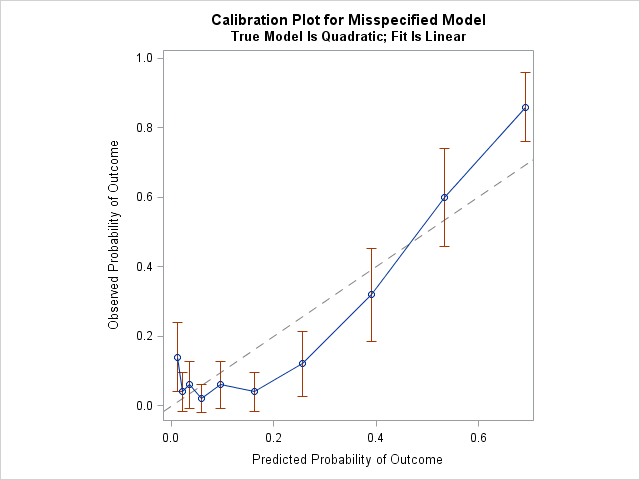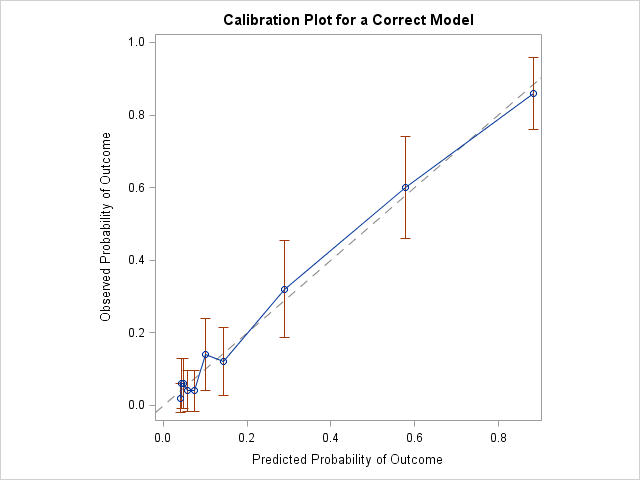In my article about how to construct calibration plots for logistic regression models in SAS, I mentioned that there are several popular variations of the calibration plot. The previous article showed how to construct a loess-based calibration curve. Austin and Steyerberg (2013) recommend the loess-based curve on the basis of an extensive simulation study. However, some practitioners prefer to use a decile calibration plot. This article shows how to construct a decile-based calibration curve in SAS.

### The decile calibration plot

The decile calibration plot is a graphical analog of the Hosmer-Lemeshow goodness-of-fit test for logistic regression models. The subjects are divided into 10 groups by using the deciles of the predicted probability of the fitted logistic model. Within each group, you compute the mean predicted probability and the mean of the empirical binary response. A calibration plot is a scatter plot of these 10 ordered pairs, although most calibration plots also include the 95% confidence interval for the proportion of the binary responses within each group. Many calibration plots connect the 10 ordered pairs with piecewise line segments, others use a loess curve or a least squares line to smooth the points.

### Create the decile calibration plot in SAS

The previous article simulated 500 observations from a logistic regression model logit(p) = b0 + b1*x + b2*x2 where x ~ U(-3, 3). The following call to PROC LOGISTIC fits a linear model to these simulated data. That is, the model is intentionally misspecified. A call to PROC RANK creates a new variable (Decile) that identifies the deciles of the predicted probabilities for the model. This variable is used to compute the means of the predicted probabilities and the empirical proportions (and 95% confidence intervals) for each decile:

```/* Use PROC LOGISTIC and output the predicted probabilities. Intentionally MISSPECIFY the model as linear. */ proc logistic data=LogiSim noprint; model Y(event='1') = x; output out=LogiOut predicted=PredProb; /* save predicted probabilities in data set */ run;   /* To construct the decile calibration plot, identify deciles of the predicted prob. */ proc rank data=LogiOut out=LogiDecile groups=10; var PredProb; ranks Decile; run;   /* Then compute the mean predicted prob and the empirical proportions (and CI) for each decile */ proc means data=LogiDecile noprint; class Decile; types Decile; var y PredProb; output out=LogiDecileOut mean=yMean PredProbMean lclm=yLower uclm=yUpper; run;   title "Calibration Plot for Misspecified Model"; title2 "True Model Is Quadratic; Fit Is Linear"; proc sgplot data=LogiDecileOut noautolegend aspect=1; lineparm x=0 y=0 slope=1 / lineattrs=(color=grey pattern=dash); *loess x=PredProbMean y=yMean; /* if you want a smoother based on deciles */ series x=PredProbMean y=yMean; /* if you to connect the deciles */ scatter x=PredProbMean y=yMean / yerrorlower=yLower yerrorupper=yUpper; yaxis label="Observed Probability of Outcome"; xaxis label="Predicted Probability of Outcome"; run;```The diagonal line is the line of perfect calibration. In a well-calibrated model, the 10 markers should lie close to the diagonal line. For this example, the graph indicates that the linear model does not fit the data well. For the first decile of the predicted probability (the lowest predicted-risk group), the observed probability of the event is much higher than the mean predicted probability. For the fourth, sixth, and seventh deciles, the observed probability is much lower than the mean predicted probability. For the tenth decile (the highest predicted-risk group), the observed probability is higher than predicted. By the way, this kind of calibration is sometimes called internal calibration because the same observations are used to fit and assess the model.

### The decile calibration plot for a correctly specified model

You can fit a quadratic model to the data to see how the calibration plot changes for a correctly specified model. The results are shown below. In this graph, all markers are close to the diagonal line, which indicates a very close agreement between the predicted and observed probabilities of the event.### Should you use the decile calibration curve?

The decile-based calibration plot is popular, perhaps because it is so simple that it can be constructed by hand. Nevertheless, Austin and Steyerberg (2013) suggest using the loess-based calibration plot instead of the decile-based plot. Reasons include the following:

• The use of deciles results in estimates that "display greater variability than is evident in the loess-based method" (p. 524).
• Several researchers have argued that the use of 10 deciles is arbitrary. Why not use five? Or 15? In fact, the results of the Hosmer-Lemeshow test "can depend markedly on the number of groups, and there's no theory to guide the choice of that number." (P. Allison, 2013. "Why I Don’t Trust the Hosmer-Lemeshow Test for Logistic Regression")

Many leading researchers in logistic regression do not recommend the Hosmer-Lemeshow test for these reasons. The decile-based calibration curve shares the same drawbacks. Since SAS can easily create the loess-based calibration curve (see the previous article), there seems to be little reason to prefer the decile-based version.

The post Decile calibration plots in SAS appeared first on The DO Loop.